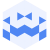Am 3. Oct. 2022 | 19:45 Uhr

# Oct 03 2022 | Tresor Linz | canceled but not canceled I Simon WhethamCreated at 3. Oct. 2022

579 Ansichten
##### by Tresor Linz

<<<<<<<<<<<<<<<<<<<<<<<<<<<<<<<<<<<<<<<<<<<<<<<<<<<<<<<<<
Simon Whetham
<<<<<<<<<<<<<<<<<<<<<<<<<<<<<<<<<<<<<<<<<<<<<<<<<<<<<<<<<
ChannellingAV is a kinetic sound performance project in which a collection of salvaged motor devices is activated by sound recordings from the environment. In turn, this produces new sounds from the devices, which are amplified using various microphones and techniques.
The recordings feature phenomena that occur unpredictably and irregularly in everyday life – passing traffic, wind, doors closing – and because of this the movement of the devices is random, variable and autonomous.

www.simonwhetham.co.uk/channellingav

<<<<<<<<<<<<<<<<<<<<<<<<<<<<<<<<<<<<<<<<<<<<<<<<<<<<<<<<<
Tresor Linz
<<<<<<<<<<<<<<<<<<<<<<<<<<<<<<<<<<<<<<<<<<<<<<<<<<<<<<<<<

Raum für Klangkunst und künstlerische Experimente.

Space for sound art and artistic experiments.

##### Share & Embed
Embed this Video